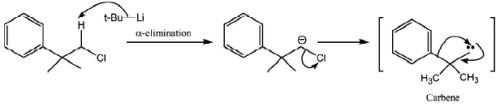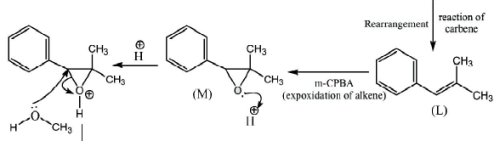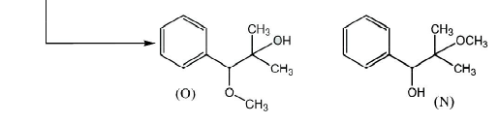Courses

# Chemistry - 2013 Past Year Paper

## 30 Questions MCQ Test IIT JAM Chemistry Past Year Papers | Chemistry - 2013 Past Year Paper

Description
This mock test of Chemistry - 2013 Past Year Paper for IIT JAM helps you for every IIT JAM entrance exam. This contains 30 Multiple Choice Questions for IIT JAM Chemistry - 2013 Past Year Paper (mcq) to study with solutions a complete question bank. The solved questions answers in this Chemistry - 2013 Past Year Paper quiz give you a good mix of easy questions and tough questions. IIT JAM students definitely take this Chemistry - 2013 Past Year Paper exercise for a better result in the exam. You can find other Chemistry - 2013 Past Year Paper extra questions, long questions & short questions for IIT JAM on EduRev as well by searching above.
QUESTION: 1

Solution:
QUESTION: 2

### Which one of the following order of the carbonates is Correct for their decomposition temperature?

Solution: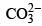is a polyatomic anion In case of polyatomic anion, Thermal stability of decomposition temperature is governed by covalent character

Thermal stability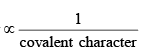Be 2+ Mg2+ Ca2+ Sr2+ Ba2+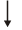Least size of cationMaximum covalent characterLeast thermally stable

∴BeCO3 < MgCO3 < CaCO3 < SrCO3 < BaCO3 Correct option is (b)

QUESTION: 3

### The Correct order of CO vibrational stretching frequency in the following complexes is (I) (PF3)3Mo(CO)3 (II) (PCl3)3Mo(CO)3 (III) {P(OMe)3}3Mo(CO)3

Solution:

Since the π-accepters attached to central metal cause the increase in the B.O. of CO, CO vibrational frequency increases. The order of π-acceptor is PF3 > PCl3 > P(OR)3 Therefore, order of vibrational stretching frequency is {P(OMe3)3} MO(CO)3 < (PCl3)3MO(CO)3 < (PF3)3MO(CO)3.
Correct option is (b)

QUESTION: 4

Among the following, the ligand that BEST stabilizes low oxidation state of tungsten (W) is

Solution:

Low oxidation state of W is stabilized by CO because of M →CO back p-bonding.
Correct option is (b)

QUESTION: 5

The function y = x exp (–x2) has a minimum at x =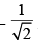The second derivative of the function at
the minimum is

Solution:

y =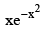minimum at x =second derivative of the function.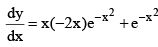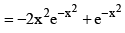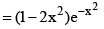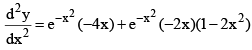Put x =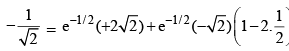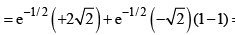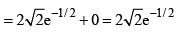QUESTION: 6

For a particular reaction at constant temperature, a plot of inverse of reactant concentration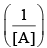versus time is a straight line with a slope of 4.0 × 10–2 L mol–1 s–1. The time required (in seconds) for 1.0 M of reactant to decrease to 0.25 M is:

Solution: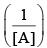vs ‘t’ graph gives straight line for a second order reaction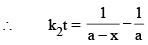... (1)

Or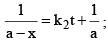y = mx + C

Slope = k2 = 4 × 10–2 L mol–1 sec–1

And a = 1.0 M      and a – x = 0.25 M

Therefore, (1), 4 × 10–2  t =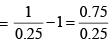⇒ t = 75

Correct option is (c)

QUESTION: 7

For a physisorption process, which one of the following statements is NOT correct?

Solution:

Physisorption is a reversible process in which the adsorbate molecules are attached to the adsorbent surface by weak vander waals forces preferably at low temperature.
Correct option is (c)

QUESTION: 8

The product of the following reaction is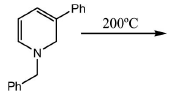Solution: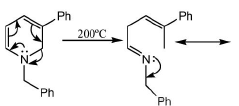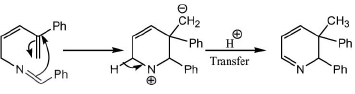QUESTION: 9

The Correct order of stability of the following carbonium ions is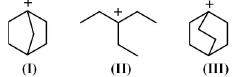Solution:

Carbocation at bridge head position is less stable because their rigid framework.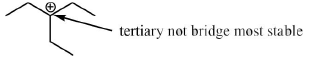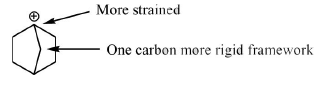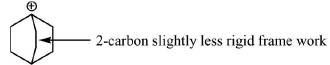So, stability of the carbocation follows the order.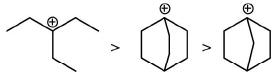Correct option is (d)

QUESTION: 10

Which one of the following statements is Correct?

Solution:

Because nucleic acids are polymer whose monomer is nucleotides not protein. So, (b) not correct.
Proteins are generally acts as enzyme but not vitamins. Proteins do not contain genetic information, its DNA which contain genetic information.
Correct option is (a)

*Answer can only contain numeric values
QUESTION: 11

The reaction of anhydrous FeCl2 with sodium-pentadienyl in ether gives an air-stable diamagnetic orange solid, which on oxidation gives an air-sensitive paramgnetic blue-green compound in solution.
The blue-green compound is _____________

Solution: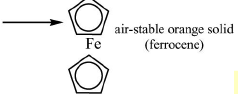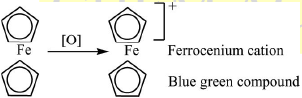*Answer can only contain numeric values
QUESTION: 12

CaO, VO and MnO have octahedral coordination of the metal ions in a rock-salt structure. The correct increasing order of their lattice enthalpies is

Solution:

CaO < MnO < VO

Because lattice energy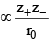QUESTION: 13

The structure of sulphur dioxide molecule (SO2) may be given as:

Solution:
*Answer can only contain numeric values
QUESTION: 14

The vapour pressures of solid and liquid chlorine are given by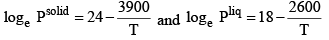where Psolid and Pliq are the vapour pressures (in Torr) of solid and liquid chlorine near the triple point, respectively and T is the absolute temperature. The ratio of the slope of the solid-gas curve to the slope of the liquid gas curve at the triple point in the P-T diagram is________

Solution:

Log P(solid ) =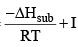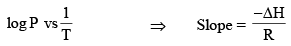Now,

Slope of sublimation curve = – 3900 Slope of vapour pressure curve = –2600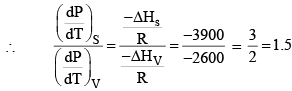*Answer can only contain numeric values
QUESTION: 15

For unnormalized wave-function ,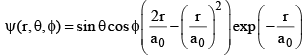the number of radial node(s) is __________

Solution:

Un normalized wave function ψ(r, θ, φ) =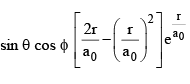Radial node is n – ℓ – 1 for given function n = 3, l = 1 Because one node at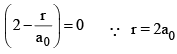Function written as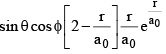Function is He+ because the part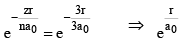Function related to 3px orbitals.

*Answer can only contain numeric values
QUESTION: 16

A hypothetical element (atomic weight = 300) crystallizes in a simple cubic lattice. For this crystal, the first order X-ray diffraction with wavelength of 5 Å appears at an angle of 30º. The density of the crystal is _____________g cm–3. [Avogadro number, NA = 6.02 × 1023]

Solution:

According to Bragg’s equation 2d sinθ = nλ ⇒ 2d sin 30 = 1 × 5 Å ⇒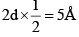⇒ d = 5Å
And for SCC, d = a = 5Å = 5 × 108 cm
Now density will be given as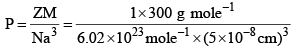= 3.9867 g cm–3

*Answer can only contain numeric values
QUESTION: 17

MnO4 (aq) + Zn(s) + H3O+ (aq) → Mn2+ (aq) + Zn2+ (aq) + H2O (ℓ) For the above reaction if the equilibrium constant at 298 K is represented by 10x, then the value of X is _________

[Given: The standard cell potential Eº = 2.4 V and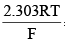= 0.06 V at 298 K]

Solution: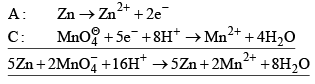n = 10
So,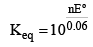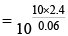x = 400

*Answer can only contain numeric values
QUESTION: 18

The rotational energy barrier between the most stable and the least stable conformations of 2, 3-dimethylbutane along C2–C3 bond is ___________kcal mol–1. [Given: The energies (kcal mol–1) for H/CH3 eclipsing = 1.8, CH3/CH3 eclipsing = 2.9 and CH3/ CH3 gauche = 0.9]

Solution: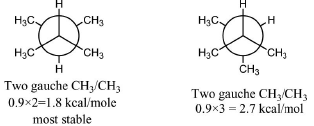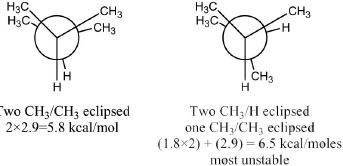Rotational energy barrier = 6.5 × 1.8 = 4.7 kcal/mol

*Answer can only contain numeric values
QUESTION: 19

The number of peaks or signals in 1H NMR of N, N-dimethylformamide (DMF) at 25ºC is ______

Solution:

Distinguishable hydrogen are indicated by a and b here. at 25ºC two signals.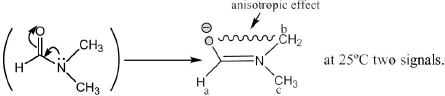*Answer can only contain numeric values
QUESTION: 20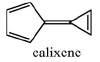Calixene is a polar hydrocarbon with a high dipole moment. The most stable dipolar canonical structure is _________

Solution: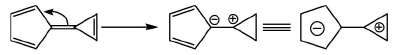Canonical

Because in this form both the rings are aromatic hence stable.

QUESTION: 21

A mixture of C3H8 and oxygen in 1L closed vessel has an internal pressure of 4 atm at 100ºC. When the mixture is ignited, the reaction produces CO2(g) and H2O(g) until all oxygen is consumed.
After the reaction, pressure of the vessel is 4.2 atm at the same temperature. Calculate the weight of oxygen present before the reaction. [Gas constant, R = 0.082 L atm mol–1 K–1].

Solution:

C3H8(g) + 5O2(g) → 3CO2(g) + 4H2O(g)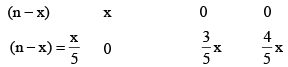Initial number of moles, n =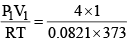= 0.1306

Final number of moles, nf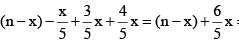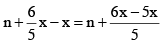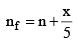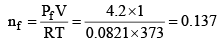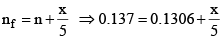x = 5 × 0.0064 ⇒ x = 0.032
weight of O2 = x × 32 = 0.032 × 32 = 1.024 gm

QUESTION: 22

The following reaction is carried out at 1 atm and 300 K 2H2(g) + O2(g) → 2H2O (l) ΔU

for the above reaction is 550 kJ. Assuming ideal gas behaviour for H2 and O2, calculate the value of ΔH. The value of gas constant, R = 0.082 L atm mol-1 K-1 = 8.314 mol-1 K-1.

[Given: The volume of 1 mole of liquid water is 18 mL under the above reaction condition]

Solution:

ΔH = ΔU + Δng RT Δng = number of gaseous moles of product - number of gaseous moles of reactant
Δng = 0 - 3 Δng = - 3 ΔU = 550 kJ
Δk = 550 kJ + (-3) × 8.314 × 300 = 550 kJ - 90058.314 = 550kJ - 7482.600 = 550 kJ - 7.4826 kJ = 542.51 kJ

QUESTION: 23

At 298 K, calculate the solubility of metal sulfide, MS(s), in a saturated solution of H2S where the concentration of H2S and pH are maintained at 0.1 M and 3.0, respectively Given at 298 K,

K = 10–7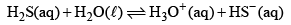K = 5 × 10–19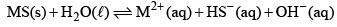Solution: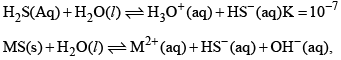K = 5 × 10–19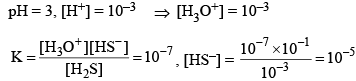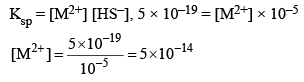Therefore, solubility of metal sulphide = 5 × 10–14 M.

QUESTION: 24

For each of the following metallo-proteins identify the metal-ion at the active-site and the function of the proteins: deoxy-hemoglobin, deoxy-myoglobin, oxy-hemocyanin, cytochrome-C and carbonic anhydrase.

Solution:

Deoxy-hemoglobin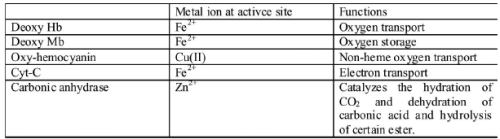QUESTION: 25

A solution containing 250 ppm of CuSO4 · 5H2O (formula weight = 250) has an absorbance of 0.1 measured in 1 cm cell at 600 nm. Calculate the molar absorptivity (e) of CuSO4 · 5H2O in L M-1 cm-1 . When 25 mL of Na2EDTA (aq) solution is titrated against Na2EDTA (aq) solution, it consumes at 50 mL of Na2EDTA (aq) solution. Calculate the concentration of Na2EDTA (aq) solution in moles L-1 .

Solution:

C = 250 ppm = 10–3 mol ltr.
A = 0.1, x = 1 cm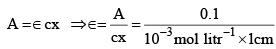= 102 litr mol-1 cm -1

QUESTION: 26

Assume the complex [NI(PPh3)2(SCN)2] is paramagnetic. The analogous complex of Pd(II) is diamagnetic. Draw all the probable isomers for both the complexes considering SCN is an ambidentate ligand.

Solution:

[Ni(PPh3)2(SCN)] → Paramagnetic, therefore, tetrahedral Isomers: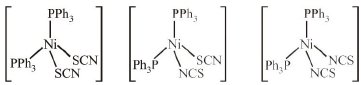[Pd(PPh3)2(SCN)2] → Diamagnetic, therefore, square planar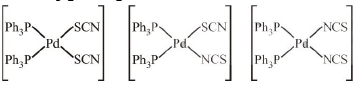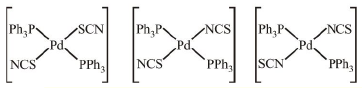QUESTION: 27

Write the structure of A to E in the following reaction sequence: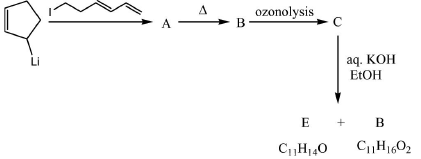Solution:

Chemical transformation involved in above chemical reaction can be illustrated as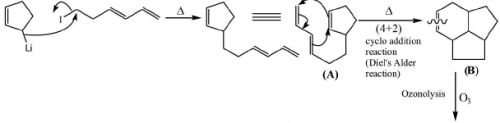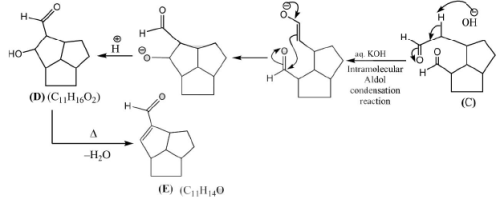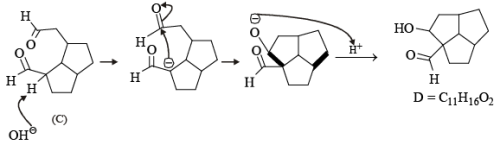QUESTION: 28

Write the structures of F to J in the following reaction scheme: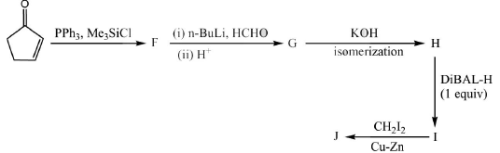[DiBAL-H = diisobutylaluminium hydride]

Solution:

Chemical transformation involved in above chemical reaction can be illustrated as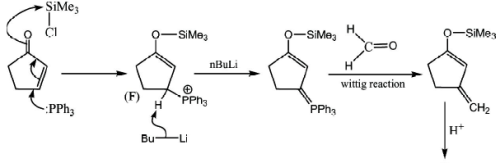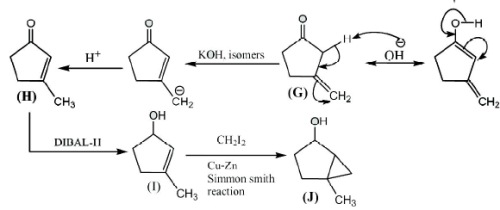QUESTION: 29

Propose a mechanism for the following reaction. Show stepwise correct reactive intermediates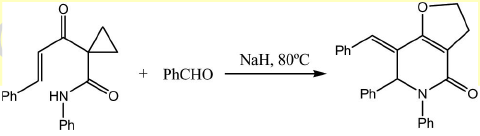Solution:

Chemical transformation involved in above chemical reaction can be illustrated as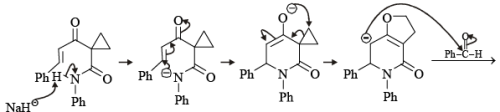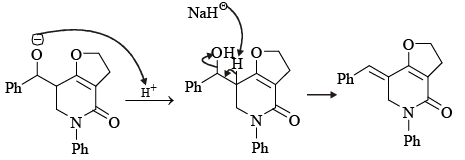QUESTION: 30

Complete the following reaction sequence and write structures of K to O.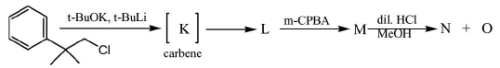Solution:

Chemical transformation involved in above chemical reaction can be illustrated as